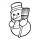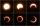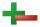# Integers + addition - math problems

#### Number of problems found: 25

• ThermometerThe thermometer showed -3°C in the morning. Then the temperature was increased by 1°C again increased by 1°C and then decreased by 1°C and then decreased by 4°C. Which terminal temperature thermometer shows?
• FractionFraction frac(0, overline(38))(0,38) write as fraction a/b, a, b is integers numerator/denominator.
• MistakeNicol mistake when calculate in school. Instead of add number 20 subtract it. What is the difference between the result and the right result?
• AVG of INTWhat is the average of the integers from 9 throuht 52 inclusive?
• Opposite numbersCalculate opposite numbers (additive inverse) to given ones:

We apologize, but in this category are not a lot of examples.
Do you have an exciting math question or word problem that you can't solve? Ask a question or post a math problem, and we can try to solve it.

We will send a solution to your e-mail address. Solved examples are also published here. Please enter the e-mail correctly and check whether you don't have a full mailbox.

Integers - math problems. Addition Problems.